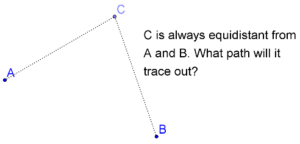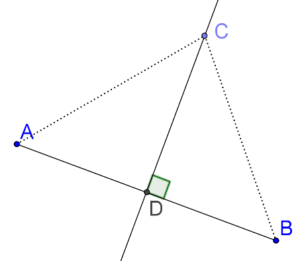# Perpendicular Bisectors

Perpendicular Bisectors
Go back to  'Triangles'

## What are perpendicular bisectors?

Consider two fixed points $$A$$ and $$B$$ in the plane. Suppose that $$C$$ is a moving point which moves in such a way so that it is always equidistant (at the same distance) from $$A$$ and $$B$$.Think: What will be the path traced out by $$C$$? In technical language, what will be the locus of $$C$$, given this constraint? Locus is a latin word, and you can think of it as the path traced out by a point under a given set of conditions.The answer to this question is: The path traced out by $$C$$ will be the perpendicular bisector of the segment joining $$A$$ and $$B$$. In other words, if $$C$$ lies anywhere on the perpendicular bisector of $$AB$$, it will necessarily be equidistant from $$A$$ and $$B$$.

Let us see how. Consider the following figure, in which $$C$$ is an arbitrary point on the perpendicular bisector of $$AB$$ (which intersects $$AB$$ at $$D$$):Compare $$\Delta ACD$$ and $$\Delta BCD$$. We have:

1. $$AD = BD$$
2. $$CD = CD$$ (common)
3. $$\angle ADC = \angle BDC = 90^\circ$$

We see that $$\Delta ACD \cong \Delta BCD$$ by the SAS congreunce criterion. Thus, $$CA = CB$$, which means that $$C$$ is equidistant from $$A$$ and $$B$$.

Note: Refer SAS congreunce criterion to understand why $$\Delta ACD$$ and $$\Delta BCD$$ are congruent.

To repeat this extremely important point once again: If a point $$C$$ is equidistant from two fixed points $$A$$ and $$B$$, then it must lie on the perpendicular bisector of $$A$$ and $$B$$. Every point on the perpendicular bisector of $$AB$$ will be equidistant from $$A$$ and $$B$$.

## Definition of Perpendicular Bisector:

perpendicular bisector is a special kind of segment, ray, or line that:

• intersects a given segment at a $$90^\circ$$ angle, and,
• passes through the given segment's midpoint.

Note: In the above figure, segment $$CD$$ is the perpendicular bisector to segment $$AB$$.

grade 9 | Questions Set 1